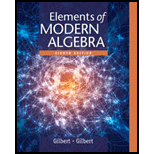# Let f : A → B , where A and B are non empty, and let T 1 and T 2 be subsets of B . Prove that f − 1 ( T 1 ∪ T 2 ) = f − 1 ( T 1 ) ∪ f − 1 ( T 2 ) . Prove that f − 1 ( T 1 ∩ T 2 ) = f − 1 ( T 1 ) ∩ f − 1 ( T 2 ) . Prove that f − 1 ( T 1 ) − f − 1 ( T 2 ) = f − 1 ( T 1 − T 2 ) . Prove that if T 1 ⊆ T 2 , t h e n f − 1 ( T 1 ) ⊆ f − 1 ( T 2 ) .### Elements Of Modern Algebra

8th Edition
Gilbert + 2 others
Publisher: Cengage Learning,
ISBN: 9781285463230### Elements Of Modern Algebra

8th Edition
Gilbert + 2 others
Publisher: Cengage Learning,
ISBN: 9781285463230

#### Solutions

Chapter
Section
Chapter 1.2, Problem 25E
Textbook Problem

## Expert Solution

### Want to see the full answer?

Check out a sample textbook solution.See solution

### Want to see this answer and more?

Experts are waiting 24/7 to provide step-by-step solutions in as fast as 30 minutes!*

See Solution

*Response times vary by subject and question complexity. Median response time is 34 minutes and may be longer for new subjects.